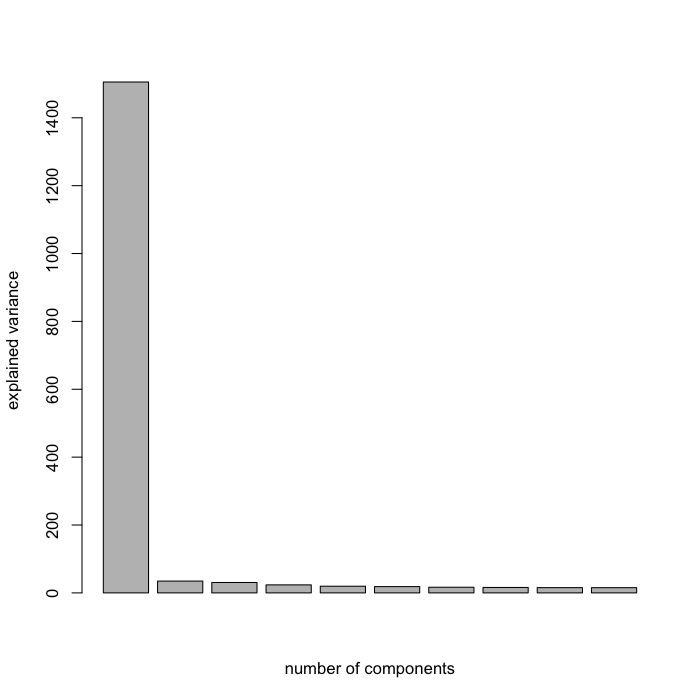# Missing values

All methodologies implemented in mixOmics can handle missing values. In particular, (s)PLS, (s)PLS-DA, (s)PCA (using the non-linear iterative partial least squares algorithm NIPALS approach) take advantage of the PLS algorithm which performs local regressions on the latent components (NIPALS, Wold 1966).

The valid() function for (s)PLS-DA has not yet been implemented to deal with missing values. A solution for now is to impute the missing values from each data set separately using the nipals() function.

# Usage in mixOmics

library(mixOmics)
data(liver.toxicity)
X <- liver.toxicity$gene[, 1:100] # a reduced size data set  ## Error in eval(expr, envir, enclos): lazy-load database '/Library/Frameworks/R.framework/Versions/3.2/Resources/library/mixOmics/data/Rdata.rdb' is corrupt  ## pretend there are 20 NA values in our data na.row <- sample(1:nrow(X), 20, replace = TRUE) na.col <- sample(1:ncol(X), 20, replace = TRUE) X.na <- as.matrix(X) ## fill these NA values in X X.na[cbind(na.row, na.col)] <- NA sum(is.na(X.na)) # Should display 20  ##  20  # this might take some time depending on the size of the data set nipals.tune = nipals(X.na, reconst = TRUE, ncomp = 10)$eig
barplot(nipals.tune, xlab = 'number of components', ylab = 'explained variance')#nipals with the chosen number of components (try to choose a large number)
nipals.X = nipals(X.na, reconst = TRUE, ncomp = 10)\$rec

#  only replace the imputation for the missing values
id.na = is.na(X.na)
nipals.X[!id.na] = X[!id.na]

nipals.X[id.na]  # imputed values

##   10.970004  4.959778  6.826238  5.142902  7.968337  5.324387  5.133662
##   10.611900  5.361598  5.257020  8.802206  5.081917  6.995585  4.711775
##   5.383448  4.982672  6.943870  5.361128  4.882336  4.640481

X[id.na]         # original values

##   11.210464  5.005651  6.839428  5.082316  7.605890  5.007477  5.242778
##   10.860053  5.139061  5.132597  8.732550  5.228111  6.096898  4.915957
##   5.009668  4.991237  7.005923  5.478554  4.952228  5.204199


# References

Wold H. (1966) Multivariate Analysis. Academic Press, New York, Wiley.

Tenenhaus M. (1998) La régression PLS : théorie et pratique. Editions Technip.